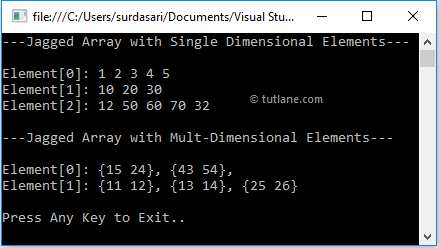# C# Jagged Arrays with Examples

In c#, Jagged Array is an array whose elements are arrays with different dimensions and sizes. Sometimes a jagged array is called an “array of arrays” and can store arrays instead of a particular data type value.

## C# Jagged Array Declaration

In c#, a jagged array can be initialized with two square brackets [][]. The first square bracket will specify the size of an array, and the second one will specify the dimension of the array, which will be stored as a value.

The following are examples of creating jagged arrays in c# programming language with single and multidimensional arrays.

// Jagged Array with Single Dimensional Array
int[][] jarray = new int[];
// Jagged Array with Two Dimensional Array
int[][,] jarray1 = new int[,];

If you observe the above examples, the first array (jarray) is allowed to store 2 elements of single-dimensional arrays. The second array (jarray1) is allowed to store 3 elements of multidimensional arrays.

## C# Jagged Array Initialization

In c#, we can initialize arrays upon declaration. The following are the different ways of declaring and initializing jagged arrays in the c# programming language.

// Jagged Array with Single Dimensional Array
int[][] jarray = new int[];
jarray = new int { 1, 2, 3, 4, 5 };
jarray = new int { 10, 20, 30 };
jarray = new int[] { 12, 50, 60, 70, 32 };
// Jagged Array with Two Dimensional Array
int[][,] jarray1 = new int[,];
jarray1 = new int[2, 2] { { 15, 24 }, { 43, 54 } };
jarray1 = new int[,] { { 11, 12 }, { 13, 14 }, { 25, 26 } };
jarray1 = new int[4, 3];
// Initializing an Array on Declaration
int[][] jarray2 = new int[][]
{
new int[]{1,2,3,4,5},
new int[]{98, 56, 45},
new int[]{32}
};

If you observe the above examples, we declared and initialized jagged arrays with single-dimensional and multidimensional arrays as elements in different ways.

In jagged arrays, the size of elements is optional. In some of the elements, we didn’t mention a size to store single or multidimensional arrays with different sizes based on our requirements.

## C# Access Jagged Array Elements

In c#, we can access jagged arrays' values by using a row index and column index values.

Following is the example of accessing elements from jagged arrays in c# programming language based on our requirements.

int i = jarray; //3
int j = jarray; //50
int k = jarray1[1, 1]; //54
int l = jarray1[2, 1]; //26

If you observe the above example, we are accessing single or multidimensional arrays by passing index values.

## C# Jagged Array Example

Following is the example of using jagged arrays in c# programming language to represent arrays as elements in an array with single or multiple dimensions.

using System;

namespace Tutlane
{
class Program
{
static void Main(string[] args)
{
// Jagged Array with Single Dimensional Array
int[][] jarray = new int[];
jarray = new int { 1, 2, 3, 4, 5 };
jarray = new int { 10, 20, 30 };
jarray = new int[] { 12, 50, 60, 70, 32 };
Console.WriteLine("---Jagged Array with Single Dimensional Elements---\n");
for (int i = 0; i < jarray.Length; i++)
{
Console.Write("Element[{0}]: ", i);
for (int j = 0; j < jarray[i].Length; j++)
{
Console.Write("{0}{1}", jarray[i][j], j == (jarray[i].Length - 1) ? "" : " ");
}
Console.WriteLine();
}
// Jagged Array with Two Dimensional Array
int[][,] jarray1 = new int[,];
jarray1 = new int[2, 2] { { 15, 24 }, { 43, 54 } };
jarray1 = new int[,] { { 11, 12 }, { 13, 14 }, { 25, 26 } };
Console.WriteLine("\n---Jagged Array with Mult-Dimensional Elements---\n");
for (int i = 0; i < jarray1.Length; i++)
{
Console.Write("Element[{0}]: ", i);
for (int j = 0; j < jarray1[i].GetLength(0); j++)
{
Console.Write("{");
for (int k = 0; k < jarray1[i].GetLength(1); k++)
{
Console.Write("{0}{1}", jarray1[i][j, k], k == (jarray1[i].GetLength(1) - 1) ? "" : " ");
}
Console.Write("{0}{1}", "}", j < jarray1.GetLength(0) ? ", " : "");
}
Console.WriteLine();
}
Console.WriteLine("\nPress Enter Key to Exit..");
}
}
}

If you observe the above example, we are creating a jagged array with single & multidimensional arrays, and we used for loop in c# to get the elements of the jagged array.

When we run the above c# program, we will get the result as shown below.If you observe the above result, we stored arrays as elements in a jagged array based on our requirements.

This is how we can use jagged arrays in our c# applications to store single or multi-dimensional arrays as an element of the jagged array.